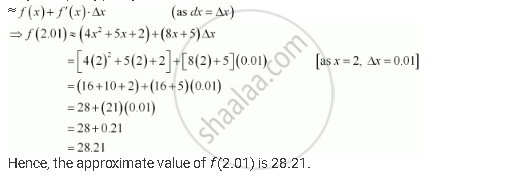Share

# Find the Approximate Value of F (2.01), Where F (X) = 4x2 + 5x + 2 - CBSE (Science) Class 12 - Mathematics

#### Question

Find the approximate value of f (2.01), where f (x) = 4x2 + 5x + 2

#### Solution

Let x = 2 and Δx = 0.01. Then, we have:

f(2.01) = f(+ Δx) = 4(x + Δx)2 + 5(x + Δx) + 2

Now, Δy = f(x + Δx) − f(x)

∴ f(x + Δx) = f(x) + ΔyIs there an error in this question or solution?

#### APPEARS IN

NCERT Solution for Mathematics Textbook for Class 12 (2018 to Current)
Chapter 6: Application of Derivatives
Q: 2 | Page no. 216

#### Video TutorialsVIEW ALL 

Solution Find the Approximate Value of F (2.01), Where F (X) = 4x2 + 5x + 2 Concept: Approximations.
S